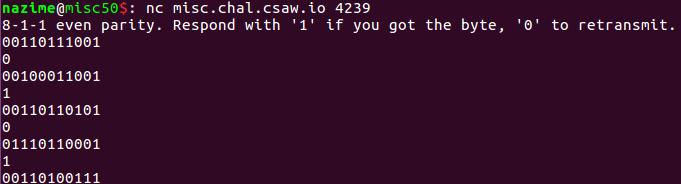# Misc 50 Serial - By Nazime

### challenge :

We are giving a server to connection via port 4239 with nc `nc misc.chal.csaw.io 4239` Connecting to the server revealed the following :
```8-1-1 even parity. Respond with '1' if you got the byte, '0' to retransmit. 00110011101```

we can respond either with 0 or with 1, and at each time we have a new sequence of bits### Analyse :

After some google search with "8-1-1 even parity" i found that two links that were usefull https://en.wikipedia.org/wiki/8-N-1 and https://learn.sparkfun.com/tutorials/serial-communication/rules-of-serial
When we send data we can have some times an error in transmition, so every time we send a byte we count the number of "1" in that byte and if the number is even we add "0" in our byte, if the number of "1" is odd we add "1" to our byte.
Exemple :
0110 1100 0 : here the number of "1" is 4 , 4 %2 == 0 , so 4 is even so the parity bit should be 0 , no error in transmition
0001 0110 0 : here the number of "1" is 3 , 3%2==1, so 3 is odd the parity bit should be 1, they have an error in transmition
0101 1110 1 : here the number of "1" is 5 , 5%2 == 1, so 5 is odd the parity number should be 1, no error in transmition
the N in 8-n-1 stand for no parity test, in our challenge we have 8-1-1 so we have a parity test in our byte.

At each time we have 11 bits, the first bit is always "0" it stand fo "start" then we have our byte (8bits) then one bit for parity then one bit who is alway "1" it stand for stop

### Solution:

The solution at this point is obvious, at each step we check if they have no error of transmition by counting the number of "1" in our byte (and only in our byte not in all 11bits) then if it correspond to our parity bit (befor the last bit) we send "1" to get the next byte, otherwise we send 0 to get the correct byte.
and we convert each byte in ascci caracter to get the flag

### Python code:

I used regular expresion to get the sequence of bits

``````import socket
import re

#nc connection
HOST = 'misc.chal.csaw.io'
PORT = 4239
client = socket.socket( socket.AF_INET, socket.SOCK_STREAM)
client.connect(( HOST, PORT ))

patterne = re.compile(r'{11}') # get 11bits
text = client.recv(8192) #  "8-1-1 even parity. Respond with '1' if you got the byte, '0' to retransmit.

flag = ""
obj = patterne.search(text)
while obj:
x = obj.group()
byte = x[1:9]
parityBit = int(x[-2]) # we convert in int, for comparision
if (byte.count("1") % 2) == parityBit : # no error of transmition we send 1
flag+= chr(int(byte,2)) #we convert our byte in int then in ascci
print flag
client.send("1\n")

else : #error of transmition we send 0
client.send("0\n")

text = client.recv(8192)
obj = patterne.search(text)

print  flag
``````

The script running :The flag is : flag{@[email protected]@_circuit-term1nating_3quipment}